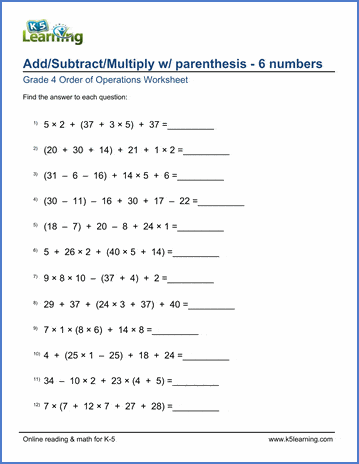# 4th Grade Order of Operations

## Order of operations worksheets

Our grade 4 order of operations worksheets provide practice in solving equations involving the 4 operations with up to 6 terms and parenthesis. No use of exponents or negative numbers.

 Add / subtract with parenthesis 33-9+40-(30+15) = Add / subtract with parenthesis (6 terms) 33-9+40+25-(30+15) = Add / subtract / multiply 3+21 x 6-(24-4) = Add / subtract / multiply  (6 terms) 3+21 x 6-(24-4) x 2 = Add / subtract / multiply / divide (62 ÷ 2 - 3) x 3 +6 = Add / subtract / multiply / divide (6 terms) (62 ÷ 2 - 3) x 3 +6 x 2=Sample Grade 4 Order of Operations Worksheet

## More order of operations worksheets

Browse all of our order of operations worksheets, from adding and subtracting with parenthesis to full PEMDAS worksheets including exponents.

What is K5?

K5 Learning offers free worksheets, flashcards and inexpensive workbooks for kids in kindergarten to grade 5. Become a member to access additional content and skip ads.